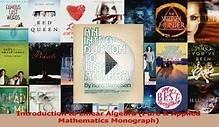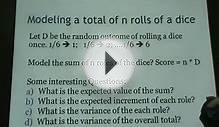# Introduction to Applied Mathematics Strang

March 17, 2023I believe that the teaching of applied mathematics needs a fresh approach. That opinion seems to be widely shared. Many of the textbooks in use today were written a generation ago, and they cannot reflect the ideas (or the algorithms) that have brought such significant change. Certainly there are things that will never be different-but even for the solution of Laplace's equation a great deal is new. -In addition to Fourier series and complex variables, it is time to see fast transforms and finite elements. Topics like stability and optimization and matrix methods have earned a more central place-perhaps at the expense of series solutions of differential equations.

Applied mathematics is alive and very vigorous. That ought to be reflected in our teaching. In my own class I became convinced that the textbook is crucial. It must provide a framework into which the applications will fit. A good course has a clear purpose, and you can sense that it is there. It is a pleasure to teach a subject when it is moving forward, and this one is-but the book has to share in that spirit and help to establish it.

The central topics are differential equations and matrix equations-the continuous and discrete. They reinforce each other because they go in parallel. On one side is calculus; on the other is algebra. Differential equations and their transforms are classical, but still beautiful and essential. Nothing is out of date about Fourier! At the same time this course must develop the discrete analogies, in which potential differences rather than derivatives drive the flow. Those analogies are not difficult; they are basic, and I have found that they are welcome. To see the cooperation between calculus and linear algebra is to see one of the best parts of modern applied mathematics.

I am also convinced that these equations are better understood-they are more concrete and useful-when we present at the same time the algorithms that solve them. Those algorithms give support to the theory. And in general, numerical methods belong with the problems they solve. I do not think that the Fast Fourier Transform and difference equations and numerical linear algebra belong only in some uncertain future course. It is this course that should recognize what the computer can do, without being dominated by it.

Perhaps I may give special mention to the role of linear algebra. If any subject has become indispensable, that is it. The days of explaining matrix notation in an appendix are gone; this book assumes a working knowledge of matrices. It begins with the solution of Ax = b - by elimination and its factorization of A into LU or LDLT, not by computing A-1. A fuller treatment is given in my textbook Linear Algebra and Its Applications (Academic Press). Here we start with the essential facts and move on rapidly to put them to use.

I do not think that the right approach is to model a few isolated examples. The important goal is to find ideas that are shared by a wide range of applications. This is the contribution a book can make, to recognize and explain the underlying pattern. We need to present Kirchhoff's laws, and to see how they lead in the continuous case to the curl and divergence, but we hope to avoid a total and fatal immersion into vector calculus-which has too frequently replaced applied mathematics, and taken all the fun out of it.

It seems natural for the discrete case to come first, but Chapter I should not be too slow. It is Chapter 2 that points out the triple product ATCA in the equations of equilibrium, and Chapter 3 finds that framework repeated by differential equations. Applications and examples are given throughout. Where the theory strengthens the understanding, it is provided-but this is not a book about proofs.

Source: www.cut-the-knot.org
##### INTERESTING VIDEORead Introduction to Linear Algebra Pure Applied ...Introduction to Mathematical Modeling for FinanceDiscrete And Combinatorial Mathematics An Applied ...